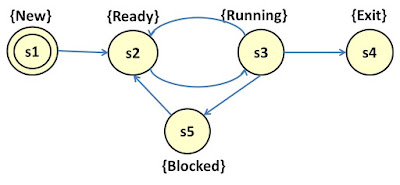# Basic Formalisms for Design Modelling

In this article we would discuss five basic formalism for design modelling. They are:

1. Kripke Structure
2. Kripke Transition System
3. Labelled Transition System
4. Finite State Automata
5. Timed Automata

2. Kripke Structure
Kripke Structure is a kind of transition system used in Model Checking to represent the behavior of a system. It is represented as a directed graph where the nodes represent the reachable states of the system and the edges represents the state transitions.
A function is used to map each of the nodes to a set of properties that hold true in that particular state

Formal Definition of Kripke Structure
Assuming AP is a set of atomic propostions (a boolean expressions over set of symbols, variable and constants), Kripke structure is defined over AP as a tuple T = {S, I, R, L} consisting of

S = {s1, s2, s2,…, sn} representing a finite set of states
I = set of initial states (subset of S)
R = set of transitions
L = labeling of the state such that LS → 2AP

Example of Kripke Structure
An example of the Kripke Structure is the process model shown in the Figure 1.Figure 1: Kripke Structure for the Process Model

Figure 1 illustrates Kripke structure for T = {S, I, R, L} where
S = { s1, s2, s3, s4, s5}
I = {s1}
R = {(s1, s2), ( s2, s3), (s3,s4), (s4,s5), (s3,s2), (s3,s5), (s5,s2)}
AP = {New, Ready, Running, Exit, Blocked}
We can also write L by mapping the transition states to the atomic propositions.

Notes on Kripke Structure
Could have more than one label per transition
Expresses properties of state and transition
Could be  used to model algorithms

3. Labelled Transition System
A labelled transition system is made up of a set of states and a set of transitions between those states. These transitions are labelled by actions. One of the states is the initial state.

Formal Definition of Labelled Transition System
A labelled transition system (LTS) over a set of actions is given by:
S = finite set of states
A = Set of actions

$\to \subseteq S×A×\mathrm{S is a set of transitions}$

$\mathrm{s0 is the initial state}$

$\mathrm{Note on Labelled Transition System}$

• $\mathrm{Has on action per transition}$
• $\mathrm{Expresses properties of transitions}$
$\mathrm{In the next part we would continue with}$
• $\mathrm{Kripke Transition System}$
• $\mathrm{Finite State Automata}$